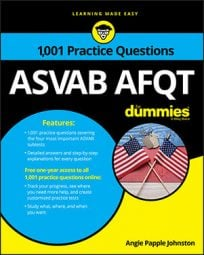##### ASVAB AFQTThe Mathematics Knowledge subtest on the ASVAB will include questions that ask you to work with parts of a whole, or fractions. These questions may involve multiplying, dividing, adding, subtracting, and converting fractions, similar to the following practice questions.

## Practice questions

1. The correct answer is Choice (C).

By finding the lowest common denominator in each set of fractions, you can tell which fractions are equal. In Choice (C), the lowest common denominator is 9. Keep the value of the fractions the same:

The two fractions in Choice (C) aren’t equal; all of the other fractions are equal.

2. The correct answer is Choice (B).

To divide fractions, you need to flip the fraction you want to divide by; then use the standard multiplication rules to work out the problem and simplify it as much as possible: